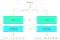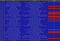# How to predict Quora Question Pairs using Siamese Manhattan LSTM

The article is about Manhattan LSTM (MaLSTM) — a Siamese deep network and its appliance to Kaggle’s Quora Pairs competition.
I will do my best to explain the network and go through the Keras code (if you are only here for the code, scroll down :)
Full code on Github

In the past few years, deep learning is all the fuss in the tech industry.
To keep up on things I like to get my hands dirty implementing interesting network architectures I come across in article readings.

Few months ago I came across a very nice article called Siamese Recurrent Architectures for Learning Sentence Similarity.It offers a pretty straightforward approach to the common problem of sentence similarity.
Named MaLSTM (“Ma” for Manhattan distance), its architecture is depicted in figure 1 (diagram excludes the sentence preprocessing part).
Notice that since this is a Siamese network, it is easier to train because it shares weights on both sides.Figure 1 MaLSTM’s architecture — Similar color means the weights are shared between the same-colored elements

# Network explained

(I will be using Keras, so some technical details are related to the implementation)

So first of all, what is a “Siamese network”?
Siamese networks are networks that have two or more identical sub-networks in them.
Siamese networks seem to perform well on similarity tasks and have been used for tasks like sentence semantic similarity, recognizing forged signatures and many more.

In MaLSTM the identical sub-network is all the way from the embedding up to the last LSTM hidden state.
Word embedding is a modern way to represent words in deep learning models. More about it can be found in this nice blog post.
Essentially it’s a method to give words semantic meaning in a vector representation.

Inputs to the network are zero-padded sequences of word indices. These inputs are vectors of fixed length, where the first zeros are being ignored and the nonzeros are indices that uniquely identify words.
Those vectors are then fed into the embedding layer. This layer looks up the corresponding embedding for each word and encapsulates all them into a matrix. This matrix represents the given text as a series of embeddings.
I use Google’s word2vec embedding, same as in the original paper.
The process is depicted in figure 2.

We have two embedded matrices that represent a candidate of two similar questions. Then we feed them into the LSTM (practically, there is only one) and the final state of the LSTM for each question is a 50-dimensional vector. It is trained to capture semantic meaning of the question.
In figure 1, this vector is denoted by the letter h.
If you don’t entirely understand LSTMs, I suggest reading this wonderful post.

By now we have the two vectors that hold the semantic meaning of each question. We put them through the defined similarity function (below)

and since we have an exponent of a negative the output (the prediction in our case) will be between 0 and 1.

# Training

This is where I will diverge a little from the original paper. For the sake of simplicity, I do not use a specific weight initialization scheme and do not pretrain it on a different task.

Other parameters such as batch size, epochs, and the gradient clipping norm value are chosen by me.

# CODE

My full implementation can be found in this Jupyter notebook — keep following this post to see only the significant parts.

Here (and in the notebook) I’ve excluded all of the data analysis part, again to keep things simple and the article readable.

# Preprocessing

We get the data as raw text, so our first mission is to take the text and convert it into lists of word indices.
When first opening the training data files in pandas, this is what you get.

Our columns of interest are question1, question2, and is_duplicate which are self-explanatory.
The training data is stored in `train_df` and the test data in `test_df` and both are pandas DataFrames.
Only difference between `train_df` and `test_df` is that the latter doesn’t have the is_duplicate column

`train_df = pd.read_csv(TRAIN_CSV)test_df = pd.read_csv(TEST_CSV)`

Next, I created a helper function named `text_to_word_list(text)` which takes a string as input and outputs a list where each entry is a single word from the text and does some preprocessing (removing specific signs etc).

Now our aim is to have the ability to turn a word into its embedding given by word2vec, in order to do that we will need to build:

• `vocabulary` which is a `dict` where the keys are words (`str`) and values are the corresponding indices (a unique id as `int`).
• `inverse_vocabulary` which is a list of words (`str`) where the index in the list is the matching id (from`vocabulary`).
We reserve the first place for an all zeros embedding — this is needed for the zero padding to be ignored.

We also use `gensim.models.KeyedVectors` to load the word2vec embeddings.
Throughout the code only 2 functions of this class will be used, `.vocab` which will hold all of the word2vec words and `.word_vec(word)` which takes a word and returns its embedding.
Finally we will use `nltk`'s English stopwords and store them in `stops`.

Creating `vocabulary` and `inverse_vocabulary`:

`vocabulary = dict()inverse_vocabulary = ['<unk>']  # '<unk>' will never be used, it is only a placeholder for the # [0, 0, ....0] embeddingword2vec = KeyedVectors.load_word2vec_format(EMBEDDING_FILE,binary=True)questions_cols = ['question1', 'question2']# Iterate over the questions only of both training and test datasetsfor dataset in [train_df, test_df]:    for index, row in dataset.iterrows():        # Iterate through the text of both questions of the row        for question in questions_cols:            q2n = []  # q2n -> question numbers representation            for word in text_to_word_list(row[question]):                # Check for unwanted words                if word in stops and word not in word2vec.vocab:                    continue                if word not in vocabulary:                    vocabulary[word] = len(inverse_vocabulary)                    q2n.append(len(inverse_vocabulary))                    inverse_vocabulary.append(word)                else:                    q2n.append(vocabulary[word])            # Replace questions with lists of word indices            dataset.set_value(index, question, q2n)`

So now we have `vocabulary`, `inverse_vocabulary` and both `train_df` and `test_df` converted to word indices, screenshot below.Figure 4 First lines of the converted to word indices training dataset.

Notice we start at index 1, index 0 is reserved for the zero padding.
Also, notice I do not exclude stopwords if they have embeddings, I will later give them a random representation — this is done for the sake of simplicity. A far better approach will be to train your own embeddings to better capture the context of the problem.

# Embedding matrix

Our next goal is to create the embedding matrix.
We will assign each word its word2vec embedding and leave the unrecognized ones (less than 0.5%) random.
Also, we keep the first index all zeros.

`embedding_dim = 300# This will be the embedding matrixembeddings = 1 * np.random.randn(len(vocabulary) + 1, embedding_dim)  embeddings = 0  # So that the padding will be ignored# Build the embedding matrixfor word, index in vocabulary.items():    if word in word2vec.vocab:        embeddings[index] = word2vec.word_vec(word)`

Great, we have our embedding matrix in place.

# Data preparation

In order to prepare our data for use in Keras we have to do two things:

• Split our data to ‘left’ and ‘right’ inputs (one for each side of the MaLSTM)
• Pad all of the word number sequences with zeros

We will also create a validation dataset, to measure our model using scikit-learn’s `train_test_split` function — it keeps the labels distribution between the datasets by default.
In `max_seq_length` we have the length of the longest question, and here is the code

`# Split to train validationvalidation_size = 40000training_size = len(train_df) - validation_sizeX = train_df[questions_cols]Y = train_df['is_duplicate']X_train, X_validation, Y_train, Y_validation = train_test_split(X, Y, test_size=validation_size)# Split to dictsX_train = {'left': X_train.question1, 'right': X_train.question2}X_validation = {'left': X_validation.question1, 'right': X_validation.question2}X_test = {'left': test_df.question1, 'right': test_df.question2}# Convert labels to their numpy representationsY_train = Y_train.valuesY_validation = Y_validation.values# Zero paddingfor dataset, side in itertools.product([X_train, X_validation], ['left', 'right']):    dataset[side] = pad_sequences(dataset[side],  maxlen=max_seq_length)`

`itertools.product` simply gives all the combinations between the two lists.

# Model

Now we create the model itself.
Most of the code is pretty clear but I would like to take a moment to talk about Keras `Merge` layer.
The `Merge` layer allows us to merge elements with some built-in methods, but also supports custom methods. This is where it comes in handy since we need to “merge” our two LSTMs output using the MaLSTM similarity function.
First let’s define the MaLSTM similarity function.

`def exponent_neg_manhattan_distance(left, right):    return K.exp(-K.sum(K.abs(left-right), axis=1, keepdims=True))`

Now lets build the model (using functional API)

`# The visible layerleft_input = Input(shape=(max_seq_length,), dtype='int32')right_input = Input(shape=(max_seq_length,), dtype='int32')embedding_layer = Embedding(len(embeddings), embedding_dim, weights=[embeddings], input_length=max_seq_length, trainable=False)# Embedded version of the inputsencoded_left = embedding_layer(left_input)encoded_right = embedding_layer(right_input)# Since this is a siamese network, both sides share the same LSTMshared_lstm = LSTM(n_hidden)left_output = shared_lstm(encoded_left)right_output = shared_lstm(encoded_right)# Calculates the distance as defined by the MaLSTM modelmalstm_distance = Lambda(function=lambda x: exponent_neg_manhattan_distance(x, x),output_shape=lambda x: (x, 1))([left_output, right_output])# Pack it all up into a modelmalstm = Model([left_input, right_input], [malstm_distance])`

Don’t you love it how simple Keras is?
Next we define the optimizer and compile our model.

`# Adadelta optimizer, with gradient clipping by normoptimizer = Adadelta(clipnorm=gradient_clipping_norm)malstm.compile(loss='mean_squared_error', optimizer=optimizer, metrics=['accuracy'])`

Now all that is left is to train it!

# Training and results

I launched it on my local machine, which has a GTX 1070.
The whole script and including preparation and training took about 21 hours.

`malstm_trained = malstm.fit([X_train['left'], X_train['right']], Y_train, batch_size=batch_size, nb_epoch=n_epoch,                            validation_data=([X_validation['left'], X_validation['right']], Y_validation),                            callbacks=[checkpointer])`

To properly evaluate the model performance, lets plot training data vs validation data accuracy and loss

Accuracy:

Loss:

So just like that out of the box, seems that MaLSTM is doing OK, getting an 82.5% accuracy rate on the validation data.

# Improvements

This article and the code as well was written with simplicity in mind.
To achieve state of the art results tuning and adjusting to your specific use case will always be needed.

Here are some thoughts of mine where can we go from here:

• Use transfer learning like in the article, to pretrain your LSTM
• Train word embeddings, on the data questions.
• Create new data. From my data exploration (is not present in this article) there are some duplicate questions that appear twice against different questions. It is possible that using this we can create more data.
• Create new data using data augmentation swapping words from the text with synonyms
• Choose a different optimizer. I’ve read a lot that Adadelta doesn’t perform as well as other methods when finely tuned.

The double-edged sword of deep learning is that this list is infinite. I’ve put there a tiny bit of possible things that keep us within the MaLSTM architecture.

If you want to explore further and to get the best results possible, I advise you to look at the discussion about the competition — they achieved some really impressive results there using various models and ensembling.

The purpose of this post was to put into work a good article that implements some things that you don’t really see in tutorials and stuff, I hope this post and the code has taught you a thing or two. Happy coding :)

Written by

Written by

## Elior Cohen

#### Data scientist, Pythonista